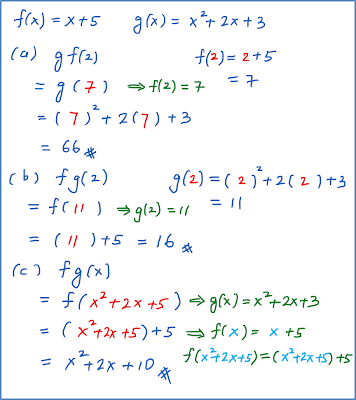1.3.1a Composite Function Example 1

Example 1
If $f:x↦x+5$ ,   and $g:x↦{x}^{2}+2x+3$  , find
(a) the value of gf(2),
(b) the value of fg(2)
(c) the composite functions fg
(d) the composite functions gf
(e) the composite functions ${g}^{2}$
(f) the composite functions ${f}^{2}$correction for part (c)correction for part (e)
$\begin{array}{l}{g}^{2}\left(x\right)=gg\left(x\right)\\ =g\left({x}^{2}+2x+3\right)\\ ={\left({x}^{2}+2x+3\right)}^{2}+2\left({x}^{2}+2x+3\right)+3\\ ={x}^{4}+2{x}^{3}+3{x}^{2}+2{x}^{3}+4{x}^{2}+6x\\ +3{x}^{2}+6x+9\\ ={x}^{4}+4{x}^{3}+10{x}^{2}+12x+9\end{array}$

3 thoughts on “1.3.1a Composite Function Example 1”

1.hiii sorry i dont understand where the 5 came for question C for composite functions

•2.